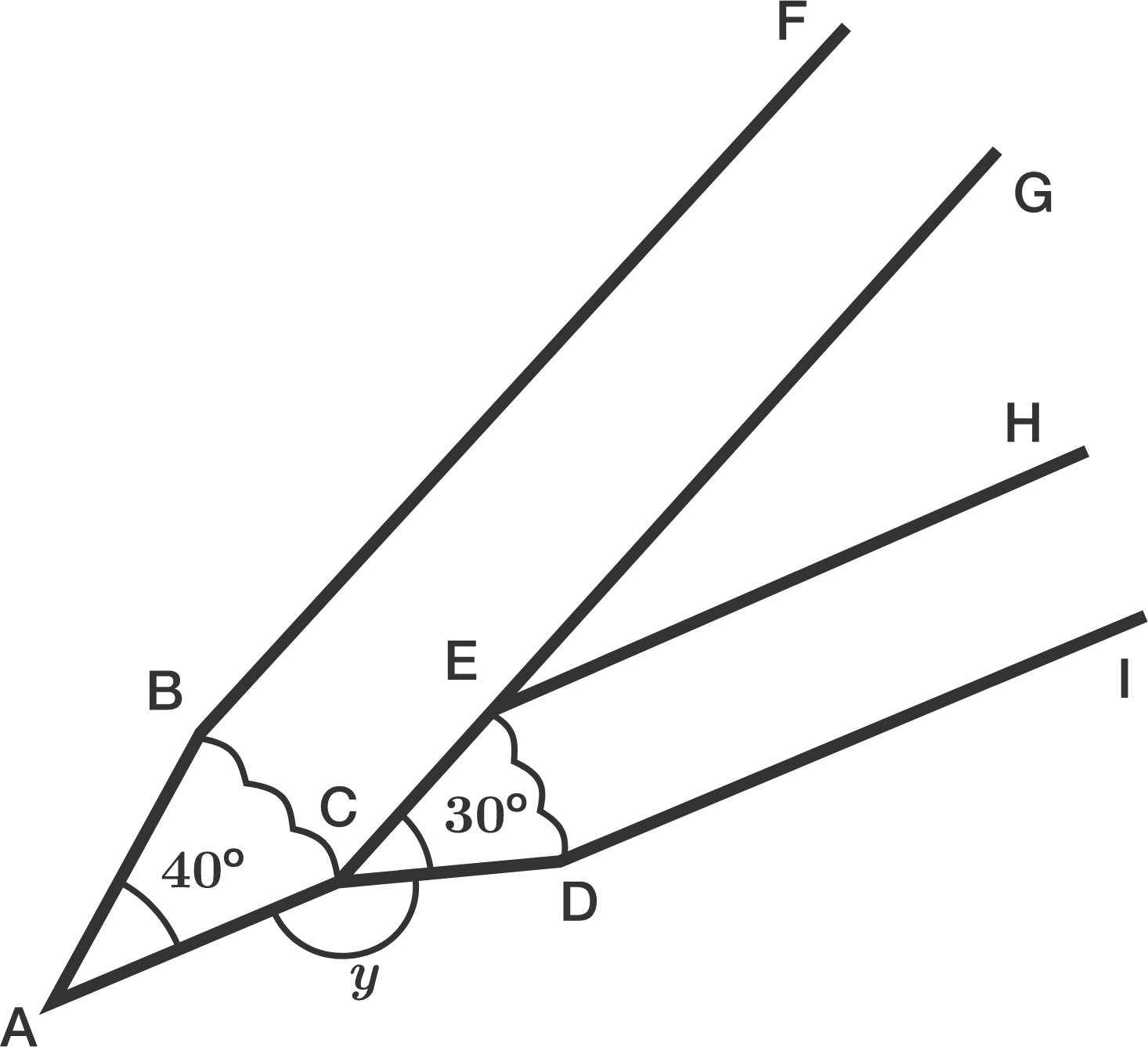# It All Came From Pencils 2

Geometry Level 1Hazri prepared another two pencils and he aligned them as shown in the diagram above.

The two pencils form an angle which is labelled $y$ ($\angle ACD$) in the diagram. If the angle of the pencil tip $A$ is $40^\circ$ and the angle of the pencil tip $ECD$ is $30^\circ$, then what is the value of $y$?

(Assume the pencils have a rectangular body and have their tips resembling isosceles triangles)

×